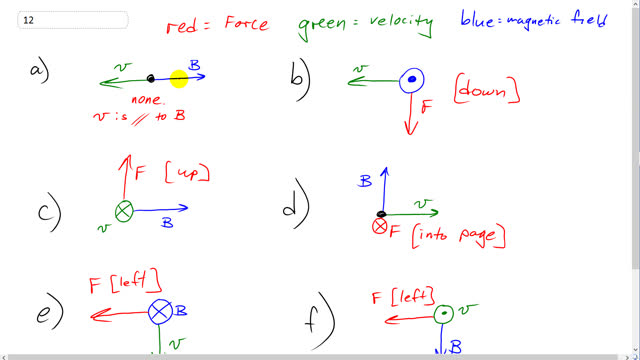## You are here

Question:

Find the direction of the force on a negative charge for each diagram shown in Fig. 20–52, where $\vec{v}$ (green) is the velocity of the charge and $\vec{B}$ (blue) is the direction of the magnetic field. ($\otimes$ means the vector points inward. $\odot$ means it points outward, toward you.)Figure 20-52 (a).Figure 20-52 (b).Figure 20-52 (c).Figure 20-52 (d).Figure 20-52 (e).Figure 20-52 (f).

Giancoli, Douglas C., Physics: Principles with Applications, 7th Ed., ©2014. Reprinted by permission of Pearson Education Inc., New York.
The question will be visible after logging in, as required by Pearson Education Inc.